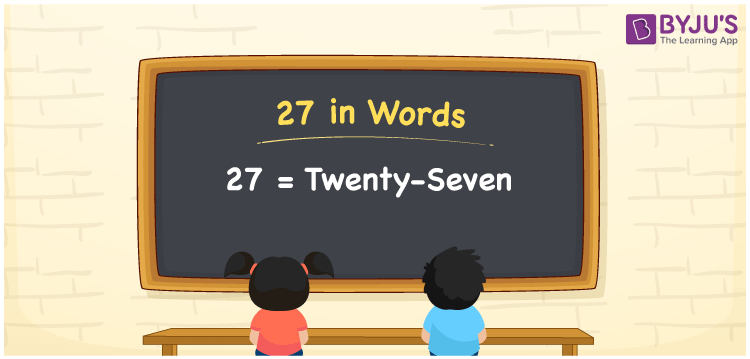# 27 in Words

27 in words is written as Twenty-seven. In both the International System of Numerals and the Indian System of Numerals, 27 in words is written as Twenty-seven. The number 27 is a Cardinal Number as it could represent some quantity. For example, “there are 27 apples in that basket”. 27 illustrates the number of apples.

 27 in Words Twenty-seven Twenty-seven in Number 27

## 27 in English Words

We write 27 in English Words using the English alphabet letters. So, we spell 27 in English as “Twenty-seven.”## How to Write 27 in Words?

To write 27 in words, we shall use the place value chart. In the place value chart, write 7 in the ones place and 2 in the tens place. Let us make a place value chart to write the number 27 in words.

 Tens Ones 2 7

Thus, we can write the expanded form as

2 × Ten + 7 × One

= 2 × 10 + 7 × 1

= 20 + 7

= 27

= Twenty-seven.

27 is the 27th natural number, the successor of 26 and the predecessor of 28.

27 in words – Twenty-seven

• Is 27 an odd number? – Yes
• Is 27 an even number? – No
• Is 27 a perfect square number? – No
• Is 27 a perfect cube number? – Yes; 33 = 27
• Is 27 a prime number? – No
• Is 27 a composite number? – Yes

## Frequently Asked Questions on 27 in Words

Q1

### How to write 27 in English words?

27 in words is written as Twenty-seven.
Q2

### How to spell 27?

The spelling of 27 is “Twenty-seven.”
Q3

### Is 27 a Whole Number?

Yes, 27 is a Whole Number.